# Using asset allocations to set account growth

An asset allocation refers to how an investment is split by underlying asset classes.  For example, we could define an investment as being made up of 70% UK Equity and 30% Fixed Interest.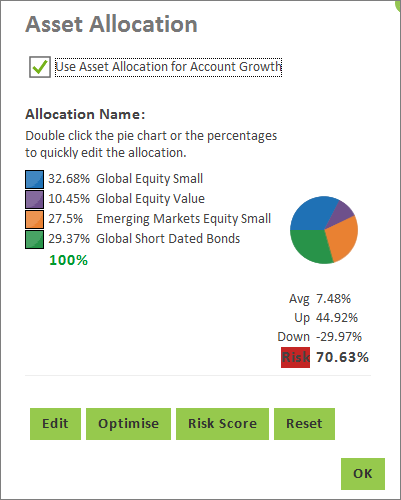Asset allocations (and the market assumptions behind them) are one of two options for driving investment returns, within the software.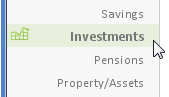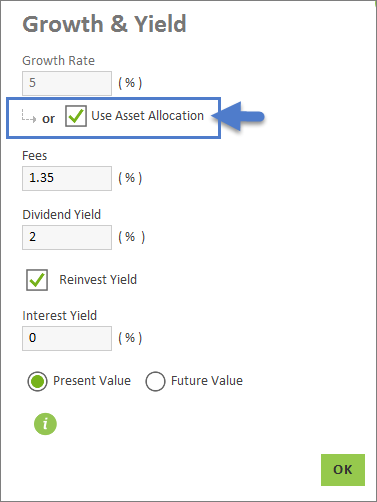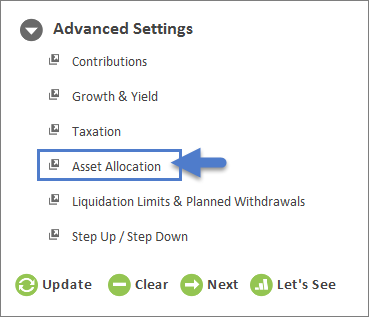The other option is using a simple fixed growth rate, as illustrated below: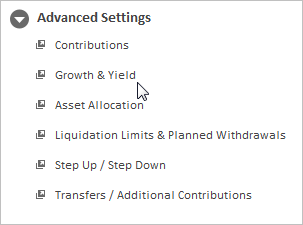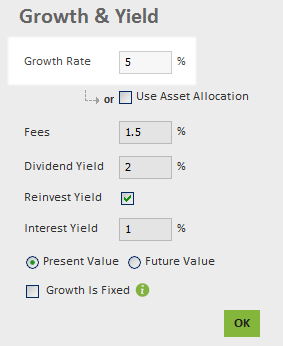Unlike a fixed growth rate, which is entirely deterministic, asset allocations permit of a range of possible returns. For normal purposes, however, the software's assumed investment return (for any given asset allocation) will correspond to the 50th percentile return (i.e. the 'mean' return). This 'mean' return will, typically, be the weighted average of the individual asset classes within the portfolio.

The software will also calculate an upside and downside range of likely returns (for a given portfolio), with each value being two standard deviations from the 50th percentile. This range of return can also be equated to a level of risk. Also note that the estimated maximum upside, and downside for a given asset allocation (i.e. portfolio) will take account of the assumed correlations between the asset classes of which the portfolio is made up, i.e. a portfolio that comprises negatively-correlated asset classes will have a lower potential upside, as well as less potential downside.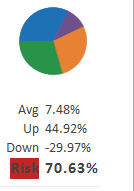When employing an asset allocation, the relevant measure of performance is relative, rather than absolute, in the sense that returns can be relatively good, or relatively bad, but the precise outcome will not be known in advance. Some of the market-based functionality within the software requires that at least one, or more accounts are to be grown using asset allocations, in order to be used. These features include the Asset Allocation Overview as well as the Monte Carlo and Historic simulations.